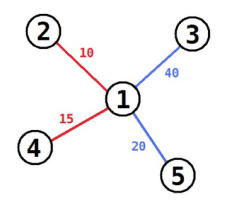# UOJ

• Append(w, v)：一个新的珠子 $w$ 和一个已经添加的珠子 $v$ 用红线连接起来。
• Insert(w, u, v)：一个新的珠子 $w$ 插入到用红线连起来的两个珠子 $u, v$ 之间。具体过程是删去 $u, v$ 之间红线，分别用蓝线连接 $u, w$ 和 $w, v$。

### 样例一

#### input

5
1 2 10
1 3 40
1 4 15
1 5 20



#### output

60



#### explanation

• 把 $5$ 和 $3$ 连起来。（线长度任意）
• 在 $3$ 和 $5$ 之间插入 $1$。（线长分别为 $40$ 和 $20$）。
• 把 $2$ 和 $1$ 用长度为 $10$ 的线连起来。
• 把 $4$ 和 $1$ 用长度为 $15$ 的线连起来。### 样例二

#### input

10
4 10 2
1 2 21
1 3 13
6 7 1
7 9 5
2 4 3
2 5 8
1 6 55
6 8 34



#### output

140



#### explanation